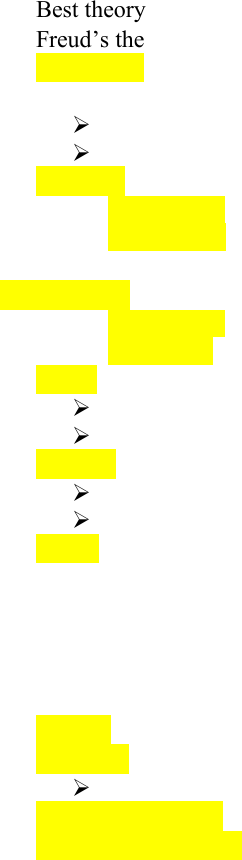Class Notes (1,100,000)
CA (630,000)
UTSC (30,000)
Psychology (8,000)
PSYA01H3 (1,000)
Lecture 2

# PSYA01H3 Lecture Notes - Lecture 2: Standard Deviation, Naturalistic Observation, Central Tendency

Department
Psychology
Course Code
PSYA01H3
Professor
Steve Joordens
Lecture
2

This preview shows half of the first page. to view the full 3 pages of the document.Chapter # 2: Reading and Evaluating Scientific Research
Lecture #5
Scientific process begins with a theory and then turns into a hypothesis: you believe to be
true
Best theory is the ones that you can find out if it is wrong
Freud’s theories are bad theories: cannot test anything
Falsifiable: shown to be wrong
Pros and cons of naturalistic observation:
Pros: find out things about the study
Cons: presence can change the behaviour of the study
Variable: is simply anything that can take multiple values (ex. Hair colour, height, etc)
Categorical: the values it can take
Continuous: the values don’t have distinct categories
Matters have the scientist codes the experiment
Statistics:
1. Descriptive: one way is to take a set of data and describe it to others clearly
2. inferential: used to answer a question
Mean: the point is the minimum possible distance from all the other points in the sample
Extreme score can have a powerful effect on the mean
Mean is not accurate in this situation
Median: the point that have the data points lie above, and half lie down
Used for central tendency
Not sensitive to outliers
Mode: the most frequently occurring data point or observation
Lecture # 6
M.A.D: the mean absolute deviation of each data point from the mean of the numbers
Variance: the average squared deviation of each data point from the mean
Squaring makes the data seem really big
Standard deviation: take the square root of the squared deviation
Correlational studies: through observation one think they see links and relations between
different variables
Positive correlation: as one variable gets higher, the other too
Negative correlation: as one variable gets higher, the other gets lower
Zero correlation: no relationship between two variables
Correlation doesn’t imply causation. Dig deeper with experiments
Lecture # 7